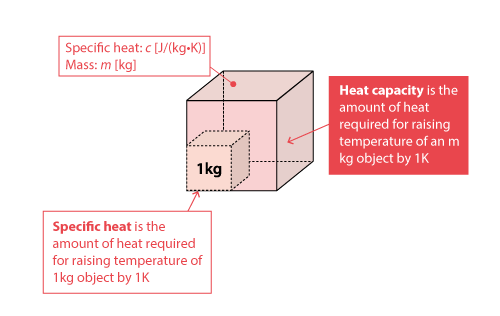#1. TOP
2. Resource Library
3. CAE Glossary
4. F-J index
5. Heat capacity

# Heat capacity

## Heat capacity

Heat capacity is the amount of heat required for raising temperature of an object by unit temperature.

It can be expressed in with the following equation:where;
: Heat capacity [J/K]
: Specific heat [J/(kg・K)]
: Mass [kg]